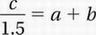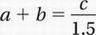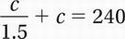# SAT Math Multiple Choice Question 775: Answer and Explanation

### Test Information

Question: 775

10. The sum of three numbers is 240. If the greatest of these numbers is 50% more than the sum of the other two, what is the value of the greatest of these numbers?

• A. 96
• B. 120
• C. 140
• D. 144

Explanation:

D

Problem Solving (rates) MEDIUM

Let's call the three numbers a, b, and c.

If their sum is 240,

a + b + c = 240

Let's say the largest number is c. If this is 50% larger than the sum of the others,

c = 1.5(a + b)

Divide by 1.5:Substituteinto the first equation:Multiply by 1.5:

c + 1.5c = 360

Simplify:

2.5c = 360

Divide by 2.5:

c = 144